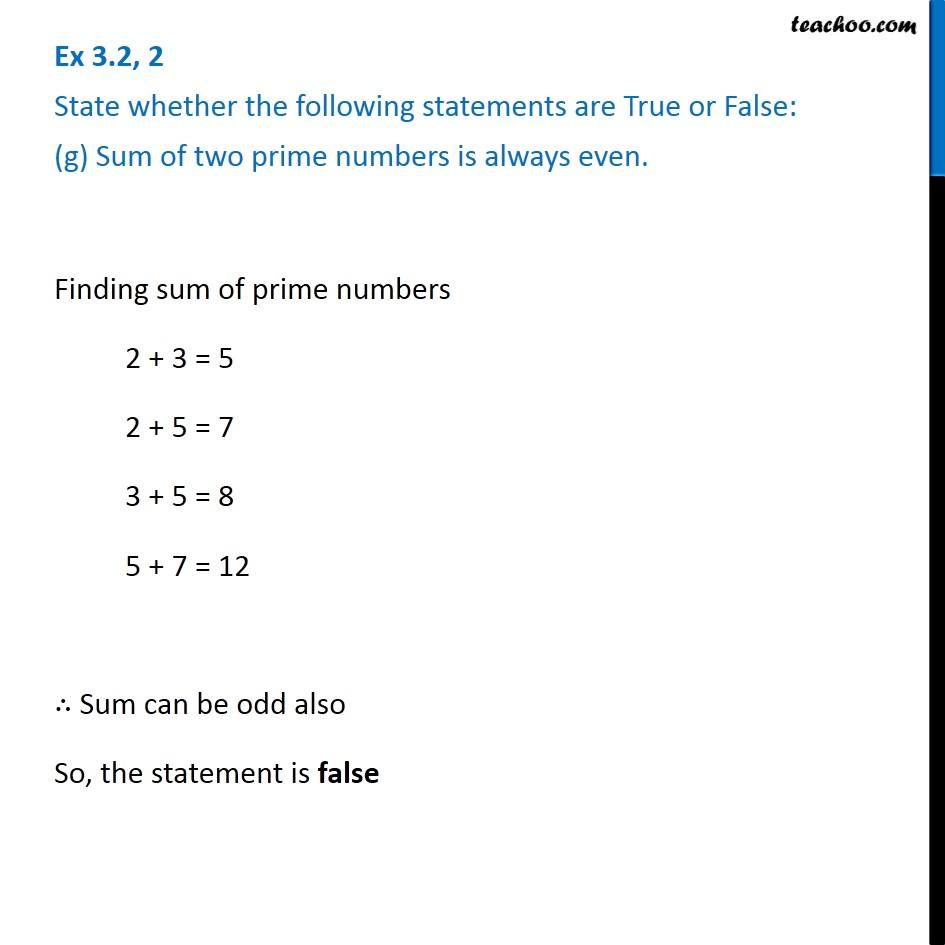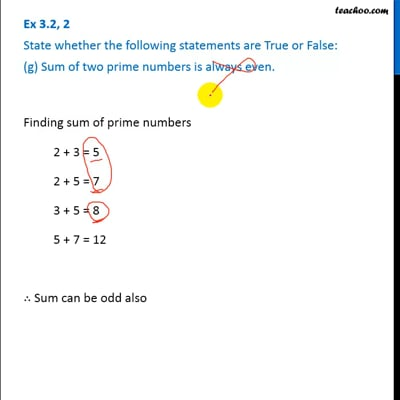Ex 3.2

Chapter 3 Class 6 Playing with Numbers
Serial order wiseThis video is only available for Teachoo black users

### Transcript

Ex 3.2, 2 State whether the following statements are True or False: (g) Sum of two prime numbers is always even. Finding sum of prime numbers 2 + 3 = 5 2 + 5 = 7 3 + 5 = 8 5 + 7 = 12 ∴ Sum can be odd also So, the statement is false